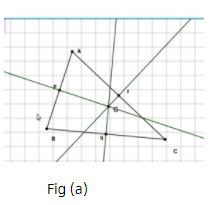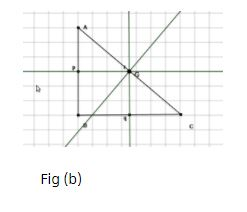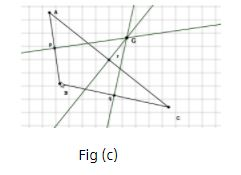# Theory - To illustrate that Perpendicular Bisectors of the Sides of a Triangle Concur at a Point Class 9 Notes | EduRev

## Class 9 : Theory - To illustrate that Perpendicular Bisectors of the Sides of a Triangle Concur at a Point Class 9 Notes | EduRev

The document Theory - To illustrate that Perpendicular Bisectors of the Sides of a Triangle Concur at a Point Class 9 Notes | EduRev is a part of the Class 9 Course Mathematics (Maths) Class 9.
All you need of Class 9 at this link: Class 9

Objective:

To illustrate that perpendicular bisectors of the sides of a triangle concur at a point(called the circumcentre) and it falls inside for an acute-angled triangle, on the hypotenuse of right-angled triangle and outside for an obtuse-angled triangle.

Definition

Circumcentre of a triangle is the point of intersection of all the three perpendicular bisectors of the triangle. It is where the "perpendicular bisectors" (lines that are at right angles to the midpoint of each side) meet. The circumcentre of a triangle is equidistant from its vertices and the distance of the circumcentre from each of the three vertices are called circum-radius of the triangle.

Properties:

1. All vertices of triangle are equidistant from circumcentre.

2. Circumcentre is also the center of circumcircle.

3. For acute angled triangle it lies inside the triangle (see fig(a)).

4. For obtuse angled triangle it lies outside the triangle (see fig(c)).

5. For right angled triangle it lies at the mid-point of hypotenuse (see fig(b)).Offer running on EduRev: Apply code STAYHOME200 to get INR 200 off on our premium plan EduRev Infinity!

## Mathematics (Maths) Class 9

190 videos|233 docs|82 tests

,

,

,

,

,

,

,

,

,

,

,

,

,

,

,

,

,

,

,

,

,

;